## Taylor Series

A Category 2 or Category 3 power series in x defines a function f by settingfor any x in the series' interval of convergence.

The power series expansion for f( x) can be differentiated term by term, and the resulting series is a valid representation of f′( x) in the same interval: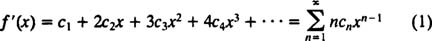Differentiating again gives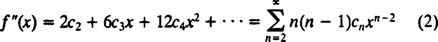and so on. Substituting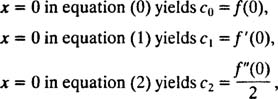and in general, substituting x = 0 in the power series expansion for the nth derivative of f yields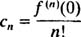These are called the Taylor coefficients of f, and the resulting power series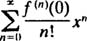is called the Taylor series of the function f.

Given a function f, its Taylor coefficients can be computed by the simple formula above, and the question arises, does the Taylor series of f actually converge to f( x)? If it does, that is, if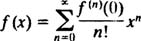for all x in some neighborhood of (interval around) 0, then the function f is said to be analytic (at 0). [More generally, if you form the Taylor series of f about a point x = x 0,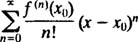and if this series actually converges to f( x) for all x in some neighborhood of x 0, then f is said to be analytic at x 0.] Polynomials are analytic everywhere, and rational functions (quotients of polynomials) are analytic at all points where the denominator is not zero. Furthermore, the familiar transcendental (that is, nonalgebraic) functions e x , sin x, and cos x are also analytic everywhere. The Taylor series in Table 1 are encountered so frequently that they are worth memorizing.

For a general power series, it is usually not possible to express it in closed form in terms of familiar functions.Example 1: Use Table 1 to find the Taylor series expansion of each of the following functions:

a.b.c. In(1 + x)

d. e x 2

e. x cos x

f. sin x cos x

g. arctan x

a. Replacing x by x 2 in the Taylor series expansion of 1/(1 – x) givessince | x| < 1 is equivalent to | x 2| < 1.

b. Differentiating 1/(1 – x) gives 1/(1 – x) 2, so differentiating the Taylor series expansion of 1/(1 – x) term by term will give the series expansion of 1/(1 – x) 2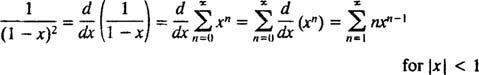c. First, replacing x by − x in the Taylor series expansion of 1/(1 – x) gives the expansion of 1/(1 + x):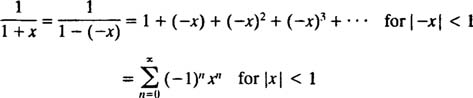Now, since integrating 1/(1 + x) yields In(1 + x), integrating the Taylor series for 1/(1 + x) term by term gives the expansion for In(1 + x), valid for | x| < 1:Technical note: Integrating 1/(1 + x) yields In (1 + x) + c (where c is some arbitrary constant), so strictly speaking, the equation above should have been writtenHowever, substituting x = 0 into this equation shows that c = 0, so the expansion given above for In (1 + x) is indeed correct.

d. Replacing x by – x 2 in the Taylor series expansion of e x yields the desired result: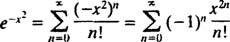e. Multiplying each term of the Taylor series for cos x by x givesf. One way to find the series expansion for sin x cos x is to multiply the expansions of sin x and cos x. A faster way, however, involves recalling the trigonometric identity sin 2 x = 2 sin x cos x and then replacing x by 2 x in the series expansion of sin x:g. Since arctan x is the integral of 1/(1 + x 2), integrate the series expansion of 1/(1 + x 2) term by term: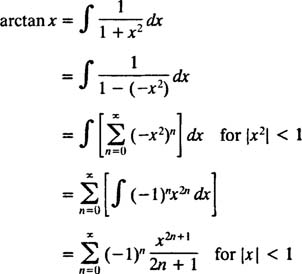Recall the technical note accompanying part c which also involved the term‐by‐term integration of a power series). The integral of 1/(1 + x 2) is actually arctan x + c, and the equation above should read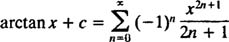However, substituting x = 0 into this equation shows that c = 0, so the expansion given above for arctan x is indeed correct.

Back to Top
A18ACD436D5A3997E3DA2573E3FD792A